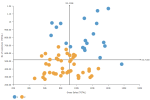# DCPY.AUTOCLUST(max_clusters, columns)

Automated clustering uses K-means algorithm to cluster the data. It starts with creating a model with two clusters and continues up to a specified maximum number of clusters, evaluating clustering quality after each model by using Calinski-Harabasz index. If the value of this index is lower than a preceding model, the preceding model is used for optimal clustering (the first local maximum of Calinski-Harabasz index).

###### Parameters
• max_clusters – The maximum number of allowed clusters, integer (default 10).

• columns – Columns to be used for clustering.

Example: DCPY.AUTOCLUST(10, sum([Gross Sales]), sum([No of customers])) used as a calculation for the Color field of the Scatterplot visualization.###### Input data
• Numeric variables are automatically scaled to zero mean and unit variance.
• Character variables are transformed to numeric values using one-hot encoding.
• Dates are treated as character variables, so they are also one-hot encoded.
• Size of input data is not limited, but many categories in character or date variables increase rapidly the dimensionality.
• Rows that contain missing values in any of their columns are dropped.
###### Result
• Column of integer values starting with 1, where each number corresponds to a cluster assigned to each record (row) by the algorithm.

• Rows that were dropped (due to missing values) are not assigned to any cluster.

###### Key usage points
• Use it when you want a quick clustering without a specific number of clusters, or without any knowledge about underlying data.

• Same assumptions and advantages as for K-means algorithm apply. For details, see DCPY.KMEANSCLUST(n_clusters, random_state, init, n_init, max_iter, columns).

• First local maximum of Calinski-Harabasz index may create clusters that are not optimal.

• Depending on the data distribution and absence of natural clusters, Calinski-Harabasz index might be often highest for the model with maximum number of clusters specified, causing also sub-optimal results.

###### Example

For the whole list of algorithms, see Data science built-in algorithms.